# How to Sort data by Column in a CSV File in Python ?

In this article, we will discuss how to sort CSV by column(s) using Python.

Method 1: Using sort_values()

We can take the header name as per our requirement, the axis can be either 0 or 1, where 0 means ‘rows’ and ‘1’ means ‘column’. Ascending can be either True/False and if True, it gets arranged in ascending order, if False, it gets arranged in descending order.

Syntax: DataFrame.sort_values(by, axis=0, ascending=True, inplace=False, kind=’quicksort’, na_position=’last’)

CSV File Used: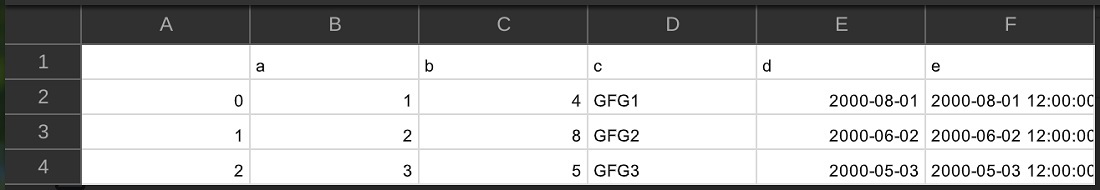Below are various which depict various ways to sort a CSV dataset.

Example 1: Sorting the dataset in descending order on the basis of salary

## Python3

 `# importing pandas package ` `import` `pandas as pandasForSortingCSV ` ` `  `# assign dataset ` `csvData ``=` `pandasForSortingCSV.read_csv(``"sample.csv"``) ` `                                        `  `# displaying unsorted data frame ` `print``(``"\nBefore sorting:"``) ` `print``(csvData) ` ` `  `# sort data frame ` `csvData.sort_values([``"Salary"``],  ` `                    ``axis``=``0``, ` `                    ``ascending``=``[``False``],  ` `                    ``inplace``=``True``) ` ` `  `# displaying sorted data frame ` `print``(``"\nAfter sorting:"``) ` `print``(csvData) `

Output: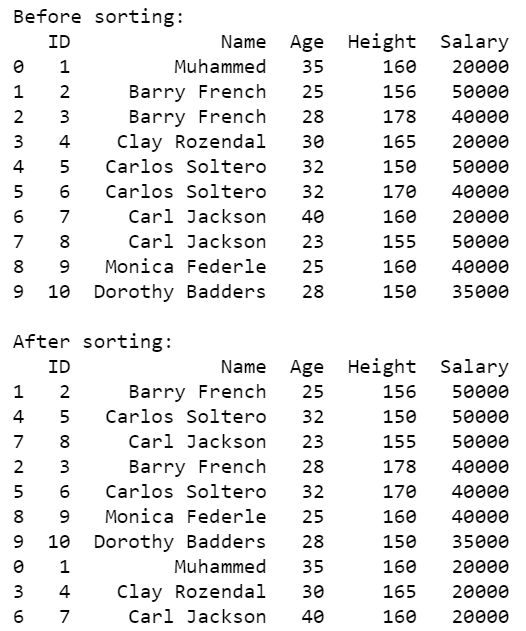Example 2: Sorting the dataset in default (ascending) order on the basis of salary.

## Python3

 `# importing pandas package ` `import` `pandas as pandasForSortingCSV ` ` `  `# assign dataset ` `csvData ``=` `pandasForSortingCSV.read_csv(``"sample.csv"``) ` `                                        `  `# displaying unsorted data frame ` `print``(``"\nBefore sorting:"``) ` `print``(csvData) ` ` `  `# sort data frame ` `csvData.sort_values(csvData.columns[``4``],  ` `                    ``axis``=``0``, ` `                    ``inplace``=``True``) ` ` `  `# displaying sorted data frame ` `print``(``"\nAfter sorting:"``) ` `print``(csvData) `

Output: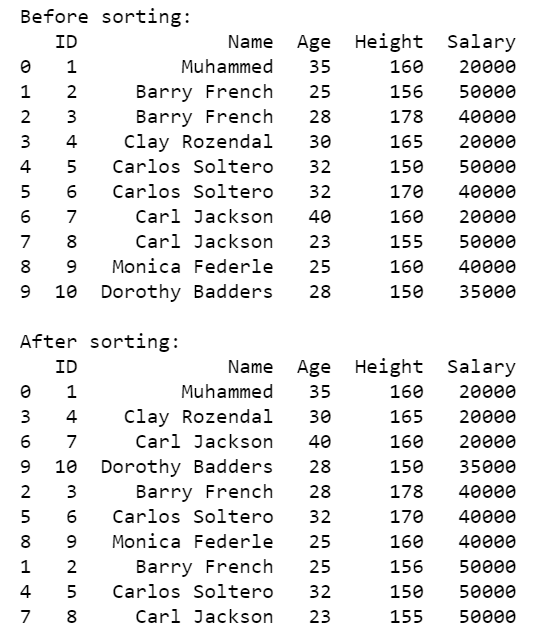Example 3: Sorting the dataset on the basis of Name, Age and, Height in ascending order.

## Python3

 `# importing pandas package ` `import` `pandas as pandasForSortingCSV ` ` `  `# assign dataset ` `csvData ``=` `pandasForSortingCSV.read_csv(``"sample.csv"``) ` `                                        `  `# displaying unsorted data frame ` `print``(``"\nBefore sorting:"``) ` `print``(csvData) ` ` `  `# sort data frame ` `csvData.sort_values([``"Name"``, ``"Age"``, ``"Height"``],  ` `                    ``axis``=``0``, ` `                    ``ascending``=``[``True``, ``True``, ``True``],  ` `                    ``inplace``=``True``) ` ` `  `# displaying sorted data frame ` `print``(``"\nAfter sorting:"``) ` `print``(csvData) `

Output: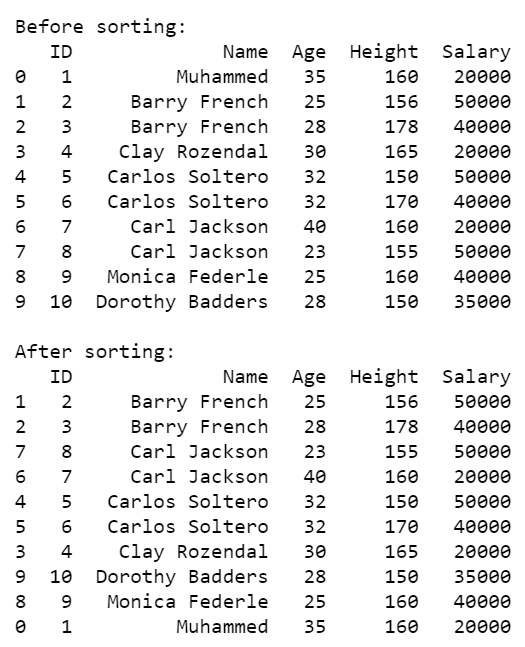Example 4: Sorting the dataset on the basis of Salary in descending order and Age in ascending order.

## Python3

 `# importing pandas package ` `import` `pandas as pandasForSortingCSV ` ` `  `# assign dataset ` `csvData ``=` `pandasForSortingCSV.read_csv(``"sample.csv"``) ` ` `  `# displaying unsorted data frame ` `print``(``"\nBefore sorting:"``) ` `print``(csvData) ` ` `  `# sort data frame ` `csvData.sort_values([csvData.columns[``4``], csvData.columns[``2``]], ` `                    ``axis``=``0``, ` `                    ``ascending``=``[``False``, ``True``], ` `                    ``inplace``=``True``) ` ` `  `# displaying sorted data frame ` `print``(``"\nAfter sorting:"``) ` `print``(csvData) `

Output: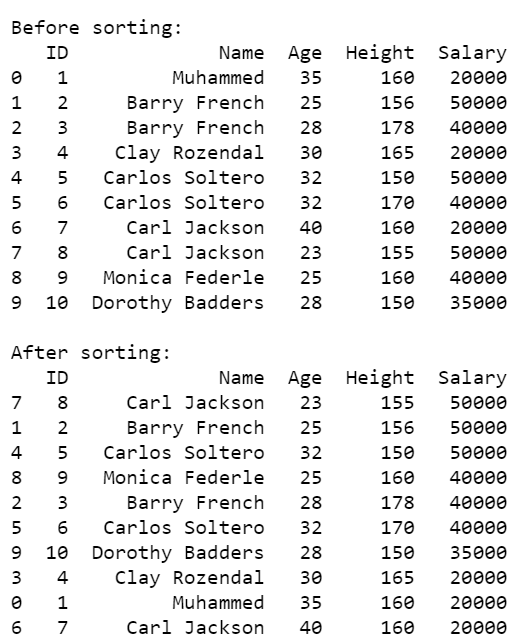Method 2: Using sorted()

Another way of sorting CSV files is by using the sorted() method on the CSV module object. However, it can only sort CSV files based on only one column.

Syntax:

sorted(iterable, key, reverse)

Below are various which depict various ways to sort a CSV dataset.

Example 1: Sorting the dataset in ascending order on the basis of Age.

## Python3

 `# import modules  ` `import` `csv ,operator ` ` `  `# load csv file ` `data ``=` `csv.reader(``open``(``'sample.csv'``),delimiter``=``','``) ` ` `  `# sort data on the basis of age ` `data ``=` `sorted``(data, key``=``operator.itemgetter(``2``))     ` ` `  `# displaying sorted data  ` `print``(``'After sorting:'``) ` `display(data) `

Output: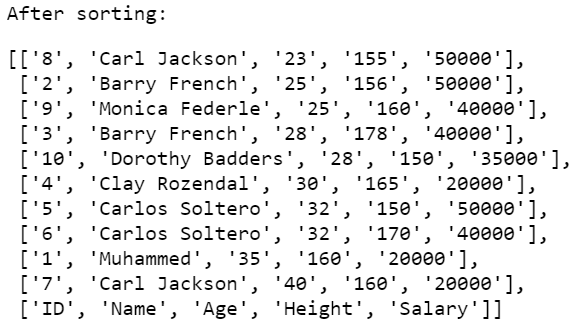Example 2: Sorting the dataset in descending order on the basis of Age.

## Python3

 `# import modules  ` `import` `csv ,operator ` ` `  `# load csv file ` `data ``=` `csv.reader(``open``(``'sample.csv'``),delimiter``=``','``) ` ` `  `# sort data on the basis of age ` `data ``=` `sorted``(data, key``=``operator.itemgetter(``2``), reverse``=``True``)     ` ` `  `# displaying sorted data  ` `print``(``'After sorting:'``) ` `display(data) `

Output: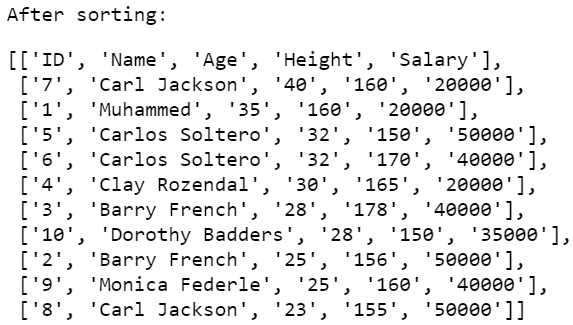Whether you're preparing for your first job interview or aiming to upskill in this ever-evolving tech landscape, GeeksforGeeks Courses are your key to success. We provide top-quality content at affordable prices, all geared towards accelerating your growth in a time-bound manner. Join the millions we've already empowered, and we're here to do the same for you. Don't miss out - check it out now!

Previous
Next